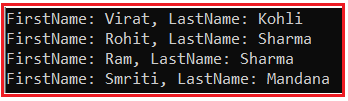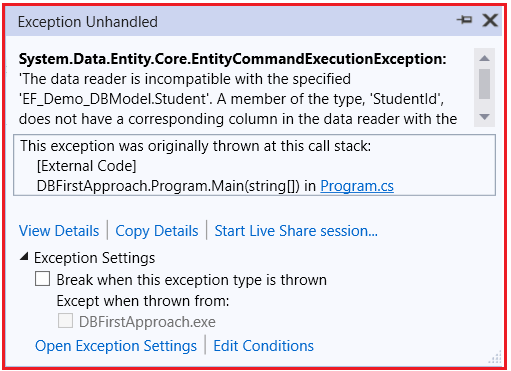# Querying in Entity Framework

## Querying in Entity Framework

In this article, I am going to discuss Querying in Entity Framework. Please read our previous article where we discussed how to perform CRUD Operations in Entity Framework Database First Approach.

Note: We are going to work with the same example that we created in our Introduction to Entity Framework Database First article. Please read our introduction to Entity Framework Database First article before proceeding to this article.

##### Different Approaches for Querying in Entity Framework

In Entity Framework, you can build and execute queries in three different ways to fetch the data from the underlying database. They are as follows:

1. LINQ-to-Entities
2. Entity SQL
3. Native SQL

#### LINQ-to-Entities Queries in Entity Framework:

The Language-Integrated Query (LINQ) is a powerful query language that was introduced with .Net 3.5 and Visual Studio 2008. You can use LINQ with C# or VB to query different types of data sources such as SQL, XML, In memory objects, etc.

As the name suggests, the LINQ-to-Entities queries operate on the entity set i.e. DbSet type properties to access the data from the underlying database. You can use both method and query syntax when querying the entities. Visit our LINQ Tutorials to learn LINQ in detail.

##### Example: LINQ-to-Entities Queries using Method syntax

In the following example, the LINQ-to-Entities query uses LINQ Method Syntax to fetches the data from the Student table where the StudentId is 1.

```using System;
using System.Linq;
namespace DBFirstApproach
{
class Program
{
static void Main(string[] args)
{
using (EF_Demo_DBEntities context = new EF_Demo_DBEntities())
{
//Fetch the studentn whose StudentId is 1 using LINQ Method Syntax
var student = context.Students.FirstOrDefault(x => x.StudentId == 1);
Console.WriteLine(\$"FirstName: {student?.FirstName}, LastName: {student?.LastName}");
}
}
}
}
```

Output: FirstName: Virat, LastName: Kohli

##### Example: LINQ-to-Entities Queries using Query syntax
```using System;
using System.Linq;

namespace DBFirstApproach
{
class Program
{
static void Main(string[] args)
{
using (EF_Demo_DBEntities context = new EF_Demo_DBEntities())
{
//Fetch the studentn whose id is 1 using LINQ Query Syntax
var student = (from std in context.Students
where std.StudentId == 1
select std).FirstOrDefault();

Console.WriteLine(\$"FirstName: {student?.FirstName}, LastName: {student?.LastName}");
}
}
}
}
```

Output: FirstName: Virat, LastName: Kohli

Note: As you can see in the above two examples, we created an instance of the context class EF_Demo_DBEntities i.e. the class which is inherited from the DbContext class. It is recommended to instantiate the DbContext class using the using() block so that once it goes out of the scope, it automatically gets disposed. In our next article, we will discuss the different ways to use LINQ-to-Entities queries.

#### Entity SQL Queries in Entity Framework

Entity SQL is another approach to write a query. It is processed by the Entity Framework’s Object Services directly. It returns ObjectQuery instead of IQueryable. In this case, you need an ObjectContext to create a query using Entity SQL. The following example shows how to use the Entity SQL Query to fetch the data from the underlying database.

```using System;
using System.Data.Entity.Infrastructure;
using System.Linq;

namespace DBFirstApproach
{
class Program
{
static void Main(string[] args)
{
using (EF_Demo_DBEntities context = new EF_Demo_DBEntities())
{
//Querying with Object Services and Entity SQL
string sqlString = "SELECT VALUE st FROM EF_Demo_DBEntities.Students " +
"AS st WHERE st.StudentId == 1";

var objctx = (context as IObjectContextAdapter).ObjectContext;
Student student  = objctx.CreateQuery<Student>(sqlString).FirstOrDefault();

Console.WriteLine(\$"FirstName: {student?.FirstName}, LastName: {student?.LastName}");
}
}
}
}
```

You can also use EntityConnection and EntityCommand to execute Entity SQL queries as shown in the below example.

```using System;
using System.Data;
using System.Data.Entity.Core.EntityClient;
namespace DBFirstApproach
{
class Program
{
static void Main(string[] args)
{
using (var con = new EntityConnection("name=EF_Demo_DBEntities"))
{
con.Open();
EntityCommand cmd = con.CreateCommand();
cmd.CommandText = "SELECT VALUE st FROM EF_Demo_DBEntities.Students as st where st.StudentId=1";
Student student = new Student();

{
{
student.StudentId = rdr.GetInt32(0);
student.FirstName = rdr.GetString(1);
student.LastName = rdr.GetString(2);
}
}

Console.WriteLine(\$"FirstName: {student?.FirstName}, LastName: {student?.LastName}");
}
}
}
}
```

Output: FirstName: Virat, LastName: Kohli

#### Native SQL Queries in Entity Framework:

The Entity Framework allows us to execute Native SQL queries for the underlying database. The following three methods are used to execute raw SQL queries in Entity Framework/

1. DbSet.SqlQuery()
2. DbContext.Database.SqlQuery()
3. DbContext.Database.ExecuteSqlCommand()
##### DbSet.SqlQuery()

The SqlQuery method on DbSet allows us to write and execute Native SQL query in Entity Framework that will return entity instances. The returned objects will be tracked by the context. The following example returns all the students from the database.

```using System;
using System.Linq;
namespace DBFirstApproach
{
class Program
{
static void Main(string[] args)
{
using (EF_Demo_DBEntities context = new EF_Demo_DBEntities())
{
//Execute Native SQL using SqlQuery Method
var studentList = context.Students
.SqlQuery("Select * from Student")
.ToList<Student>();

foreach(var student in studentList)
{
Console.WriteLine(\$"FirstName: {student?.FirstName}, LastName: {student?.LastName}");
}

}
}
}
}
```

In the above example, the Native SQL Query “Select * from Student” will execute in the database to get all students, and then it will be converted into a list of Student entities. The column names in the SQL query must match with the properties of an entity type, otherwise, it will throw an exception. When you execute the above program, you will get the following output.You can specify the parameters using the object of SqlParameter, as shown in the below example.

```using System;
using System.Data.SqlClient;
using System.Linq;

namespace DBFirstApproach
{
class Program
{
static void Main(string[] args)
{
using (EF_Demo_DBEntities context = new EF_Demo_DBEntities())
{
var student = context.Students
.SqlQuery("Select * from Student where StudentId=@StudentId", new SqlParameter("@StudentId", 1))
.FirstOrDefault();

Console.WriteLine(\$"FirstName: {student?.FirstName}, LastName: {student?.LastName}");

}
}
}
}
```

Note: If you change the column name in the SQL query, then it will throw an exception because the column names must be matched with entity properties. The following example will throw an exception.

```using System;
using System.Data.SqlClient;
using System.Linq;
namespace DBFirstApproach
{
class Program
{
static void Main(string[] args)
{
using (EF_Demo_DBEntities context = new EF_Demo_DBEntities())
{
var student = context.Students
.SqlQuery("Select StudentId as Id, FirstName, LastName, StandardId as STDID from Student where StudentId=@StudentId", new SqlParameter("@StudentId", 1))
.FirstOrDefault();

Console.WriteLine(\$"FirstName: {student?.FirstName}, LastName: {student?.LastName}");

}
}
}
}
```
###### Output:The DbSet<TEntity>.SqlQuery() method in Entity Framework executes only the Native SQL for the table which is mapped with the specified entity and only returns the result from the corresponding database table and not from any other table. The following will throw an exception.

```using System;
using System.Linq;
namespace DBFirstApproach
{
class Program
{
static void Main(string[] args)
{
using (EF_Demo_DBEntities context = new EF_Demo_DBEntities())
{
var student = context.Students
.SqlQuery("Select * from Course")
.FirstOrDefault();

Console.WriteLine(\$"FirstName: {student?.FirstName}, LastName: {student?.LastName}");

}
}
}
}
```

In the above example, the DbSet class Student is used and we are trying the access the data from the Course. And hence when you execute the above program, you will get the following exception.###### SQL Query for Non-entity Types

The SQL query returning instances of any type, including the primitive types, can be created using the SqlQuery method on the Database class. For example, in the code, we are returning only the FirstName which is of type string.

```using System;
using System.Data.SqlClient;
using System.Linq;
namespace DBFirstApproach
{
class Program
{
static void Main(string[] args)
{
using (EF_Demo_DBEntities context = new EF_Demo_DBEntities())
{
//Get student name of string type
string studentName = context.Database.SqlQuery<string>("Select FirstName from Student where StudentId=1")
.FirstOrDefault();
//or
//string studentName = context.Database.SqlQuery<string>("Select FirstName from Student where StudentId=@StudentId", new SqlParameter("@StudentId", 1))
//                        .FirstOrDefault();

Console.WriteLine(\$"studentName: {studentName}");
}
}
}
}
```
##### Database.SqlQuery()

The Database class represents the underlying database and provides various methods to deal with the database. The Database.SqlQuery() method returns a value of any type.

```using System;
using System.Data.SqlClient;
using System.Linq;
namespace DBFirstApproach
{
class Program
{
static void Main(string[] args)
{
using (EF_Demo_DBEntities context = new EF_Demo_DBEntities())
{
//Get student name of string type
var student = context.Database.SqlQuery<Student>("Select * from Student where StudentId = 1")
.FirstOrDefault();

//or
//string student = context.Database.SqlQuery<string>("Select * from Student where StudentId=@StudentId", new SqlParameter("@StudentId", 1))
//                        .FirstOrDefault();

Console.WriteLine(\$"FirstName: {student?.FirstName}, LastName: {student?.LastName}");
}
}
}
}
```

Output: FirstName: Virat, LastName: Kohli

##### Database.ExecuteSqlCommand()

The Database.ExecuteSqlCommnad() method is useful in executing database DML commands, such as the Insert, Update, and Delete commands.

```using System;
namespace DBFirstApproach
{
class Program
{
static void Main(string[] args)
{
using (EF_Demo_DBEntities context = new EF_Demo_DBEntities())
{
int noOfRowUpdated = context.Database.ExecuteSqlCommand("Update Student set FirstName = 'FirstName Changed' where StudentId = 1");
int noOfRowInserted = context.Database.ExecuteSqlCommand("Insert into Student(FirstName, LastName, StandardId) values('F1', 'L1', 1)");
int noOfRowDeleted = context.Database.ExecuteSqlCommand("Delete from Student  where Studentid = 7");